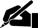# Data Analysis Chapter: Correlation and Regression

Pages: 3 (884 words)  ·  Bibliography Sources: 1  ·  Level: Master's  ·  Topic: Education - Mathematics  ·  Buy This Paper

SPSS Statistics: Correlation & Regression

Correlation & Regression

Is there a relationship between defect rate and volume? If so, is it positive or negative?

Yes, there is a relationship between defect rate and volume. The relationship is positive, such that as volume increases, so does the defect rate (.740).

Which variable is the independent and which is the dependent variable?

The independent variable (predictor) is the volume of production, and the dependent variable is the defect rate (outcome).

Write out the regression equation and sketch it on the plot.

Predicted score = Bslope X + Bconstant

Predicted score = 0.027(X) + (-97.073)

Based on a review of the plot provided, and examining two points -- 4400 and 4000, which respectively appear to hit the Y axis at 10% and 20.7%, the slope can be calculated to be

Thus, the regression equation would be:

Predicted Score = 0.027 (X) --

54% of the variability in defect rate can be explained by differences in volume.

5. What defect rate would you predict for a shift with a volume of 4000 units?

Defect Rate = 0.027 (4000) -- 97.073

= 10.927

6. What defect rate would you predict for a shift with a volume of 9000 units?

Defect Rate = 0.027 (9000) -- 97.073

= 145.927

7. Would you expect all shifts that produced 4000 items to have the same defect rate?

No. There can still be variance.

8. What would you estimate the standard deviation of the distribution of the defect rate to be for a volume of 4000 units?

The standard deviation of the intercept is 7.819

The standard deviation of the slope is .002

The Standard Error of the Estimate is 4.92.

9. If a particular shift produced 4000 items and had a defect rate of 10%, based on the regression model what would be the residual for the shift?

-.927, as the actual defect rate is .927 below the predicted defect rate based on this model.

Question 11B

1. Yes there appears to be a linear relationship between husband and wife's education.

2. The relationship between husband and wife's education appears to be positive, such that as one increases, so does the other.

3. The slope is .620 -- such that for every unit of increase in husband's education, the wife's education increases by .62.

4. The correlation coefficient (beta) is .561.

5. There are a few outliers on the scatterplot. In most of these cases they represent husbands who have higher educations than their wives.

Question 11C

1. Husband's education = .620(X)+5.341

31.4% of the variability in husband's education can be explained by wife's education.

2. Husband's education = .620(13)+5.341… [END OF PREVIEW]

### Ordering Options:

or

2.  Let us write a NEW paper for you!Most popular!

#### Correlation and Regression Research Proposal…

Cite This Data Analysis Chapter:

APA Format

Correlation and Regression.  (2010, June 29).  Retrieved September 17, 2019, from https://www.essaytown.com/subjects/paper/correlation-regression/6803924

MLA Format

"Correlation and Regression."  29 June 2010.  Web.  17 September 2019. <https://www.essaytown.com/subjects/paper/correlation-regression/6803924>.

Chicago Format

"Correlation and Regression."  Essaytown.com.  June 29, 2010.  Accessed September 17, 2019.
https://www.essaytown.com/subjects/paper/correlation-regression/6803924.